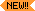### Classic VA FileMan API

This entry point is similar to ^DIC and IX^DIC, except for the way it uses cross-references to do lookup. The three entry points perform lookups as follows:

 ^DIC Starts with the B cross-reference or uses only the B cross-reference (unless K is passed in DIC(0)). IX^DIC Starts with the cross-reference you specify or uses only the cross-reference you specify. MIX^DIC1 Uses the set of cross-references you specify.
##### Input Variables (Required)

NOTE: All of the input variables described in ^DIC can be used in the MIX^DIC1 call. The following variables are required.

 DIC The global root of the file, e.g., ^DIZ(16000.1,. DIC(0) The lookup parameters as previously described for ^DIC. D The list of cross-references, separated by up-arrows, to be searched, e.g., D="SSN^WARD^B". If DIC(0) contains "L", meaning that the user can add a new entry to the file, then either a) the "B" index should be included in the list contained in D, or b) D should be set to the name of a compound index. Make sure DIC(0) contains M; otherwise, only the first cross-reference in D will be used for the lookup. X If DIC(0) does not contain an A, then the variable X must be defined equal to the value you want to look up. If the lookup index is compound (i.e., has more than one data subscript), then X can be an array X(n) where "n" represents the position in the subscript. For example, if X(2) is passed in, it will be used as the lookup value to match to the entries in the second subscript of the index. If only the lookup value X is passed, it will be assumed to be the lookup value for the first subscript in the index, X(1).##### Input Variables (Optional)

All of the ^DIC input variables can be used in the MIX^DIC1 call. These variables below are optional.

 DIC("A"), DIC("B"), DIC("DR"), DIC("P"), DIC("PTRIX",f,p,t)=d DIC("S"), DIC("V"), DIC("W") DIC("?N",file#)=n This set of input variables affects the behavior of lookup as described for ^DIC.
##### Output Variables
 Y DIC always returns the variable Y. The variable Y is returned in one of the three following formats: Y=-1 The lookup was unsuccessful. Y=N^S N is the Internal Entry Number of the entry in the file and S is the value of the .01 field for that entry. Y=N^S^1 N and S are defined as above and the 1 indicates that this entry has just been added to the file. Y(0) This variable is only set if DIC(0) contains a Z. When the variable is set, it is equal to the entire zero node of the entry that was selected. Y(0,0) This variable also is only set if DIC(0) contains a Z. When the variable is set, it is equal to the external form of the .01 field of the entry. The following are examples of returned Y variables based on a call to the EMPLOYEE file stored in ^EMP(: ``` S DIC="^EMP(",DIC(0)="QEZ",X="SMITH" D ^DIC ``` Returned are: ``` Y = "7^SMITH,SAM" Y(0) = "SMITH,SAM^M^2231109^2 Y(0,0) = "SMITH,SAM" ``` If the lookup had been done on a file whose .01 field points to the EMPLOYEE file, the returned variables might look like this: ``` Y = "32^7" [ Entry #32 in this file and #7 in EMPLOYEE file.] Y(0) = "7^RX 2354^ON HOLD" Y(0,0) = "SMITH,SAM" [.01 field of entry 7 in EMPLOYEE file] ``` X Contains the value of the field where the match occurred. If the lookup index is compound (i.e., has more than one data subscript), and if DIC(0) contains an A so that the user is prompted for lookup values, then X will be output as an array X(n) where "n" represents the position in the subscript and will contain the values from the index on which the entry was found. Thus, X(2) would contain the value of the second subscript in the index. If possible, the entries will be output in their external format (i.e., if the subscript is not computed and doesn't have a transform). If the entry is not found on an index (for example, when lookup is done with X=" " [the space-bar return feature]), then X and X(1) will contain the user input, but the rest of the X array will be undefined.DTOUT This is only defined if DIC has timed-out waiting for input from the user. DUOUT This is only defined if the user entered an up-arrow.

Reviewed/Updated: March 4, 2007